# Explicit formulas for Euler polynomials and Bernoulli numbers

Laala Khaldi, Farid Bencherif and Miloud Mihoubi
Notes on Number Theory and Discrete Mathematics
Print ISSN 1310–5132, Online ISSN 2367–8275
Volume 27, 2021, Number 4, Pages 80—89
DOI: 10.7546/nntdm.2021.27.4.80-89

## Details

### Authors and affiliations

Laala KhaldiDepartment of Mathematics, University of Bouira,
10000 Bouira, Algeria
Laboratory EDPNL&HM, Department of Mathematics,
ENS, BP 92, Vieux-Kouba, Algeria

Farid BencherifLaboratory LA3C, Faculty of Mathematics, USTHB
BP 32, El Alia , 16111, Algiers, Algeria

Miloud MihoubiLaboratory RECITS, Faculty of Mathematics, USTHB
BP 32, El Alia, 16111, Algiers, Algeria

### Abstract

In this paper, we give several explicit formulas involving the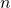-th Euler polynomial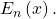For any fixed integer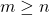, the obtained formulas follow by proving that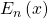can be written as a linear combination of the polynomials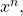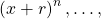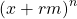, with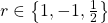. As consequence, some explicit formulas for Bernoulli numbers may be deduced.

### Keywords

• Appell polynomials
• Euler polynomials
• Bernoulli numbers
• Binomial coefficients

• 11B68
• 05A10

### References

1. Abramowitz, M., & Stegun, I. A. (1964). Handbook of Mathematical Functions, National Bureau of Standards.
2. Appell, P. (1880). Sur une classe de polynomes, Annales Scientifiques de l’Ecole Normale Superieure, 9(2), 119–144.
3. Carlitz, L. (1953). Remark on a formula for the Bernoulli numbers, Proceedings of the American Mathematical Society, 4, 400–401.
4. Garabedian, H. L. (1940). A new formula for the Bernoulli numbers, Bulletin of the American Mathematical Society, 46(6), 531–533.
5. Gould, H. W. (1972). Explicit formulas for Bernoulli numbers, The American Mathematical Monthly, 79(1), 44–51.
6. Gould, H. W. (1972). Combinatorial Identities, revised edition, Morgantown, West-Virginia.
7. Khaldi, L., Bencherif, F., & Derbal, A. (in press). A note on explicit formulas for Bernoulli polynomials. Journal of Siberian Federal University. Mathematics & Physics.
8. Quaintance, J., & Gould, H. W. (2016). Combinatorial Identities for Stirling Numbers: The Unpublished of H. W. Gould, World Scientific Publishing Co. Pte. Ltd, available at: https://www.abebooks.fr/9789814725262/CombinatorialIdentities-Stirling-Numbers-Unpublished-9814725269/plp
9. Robert, A. M. (2000). A Course in p-adic Analysis, Springer-Verlag, New York.
10. Rzadkowski, G. (2004). A Short Proof of the Explicit Formula for Bernoulli Numbers, The American Mathematical Monthly, 111(5), 433–435.
11. Worpitzky, J. (1883). Studien uber die Bernoullischen und Eulerschen Zahlen,  Journal fur die reine und angewandte Mathematik, 94, 203–232.

## Cite this paper

Khaldi, L., Bencherif, F., & Mihoubi, M. (2021). Explicit formulas for Euler polynomials and Bernoulli numbers. Notes on Number Theory and Discrete Mathematics, 27(4), 80-89, doi: 10.7546/nntdm.2021.27.4.80-89.